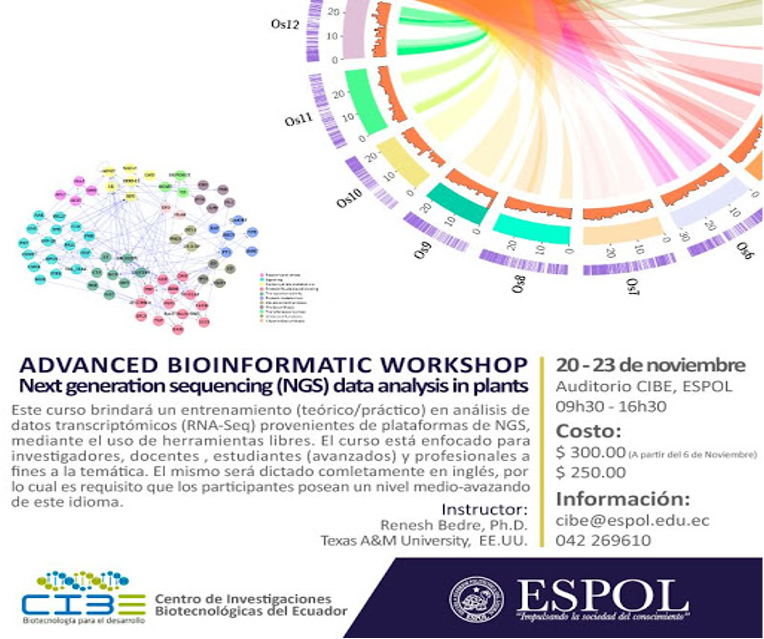# Make discoveries with your data

## Chi-square (χ2) test in Python (Pearson Chi-square test)

Pearson Chi-square test, chi-square goodness of fit test, formula, assumptions, example in Python, and interpretation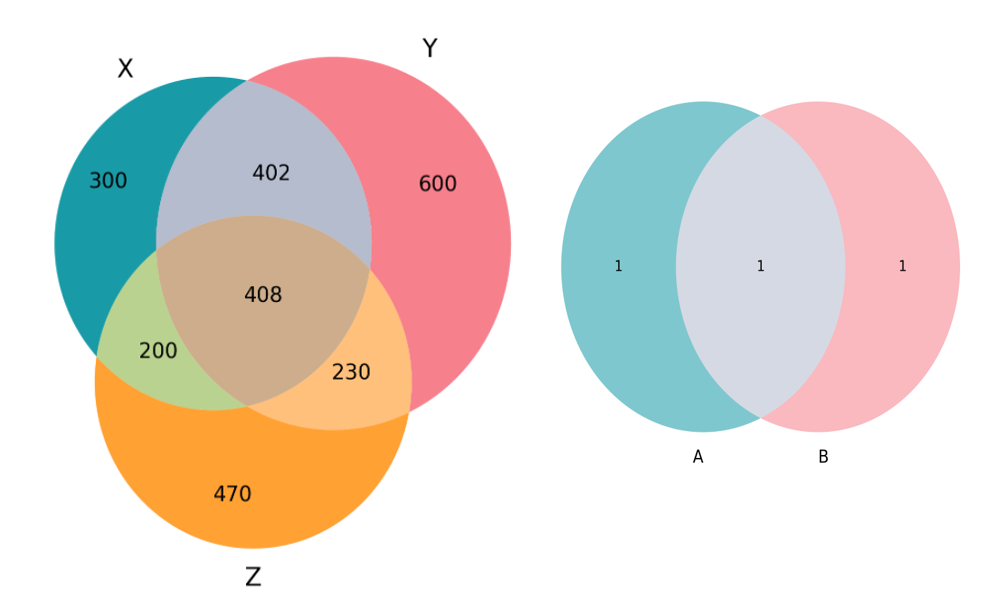## Venn diagrams in Python and R

Create two and three-way Venn diagrams in Python and R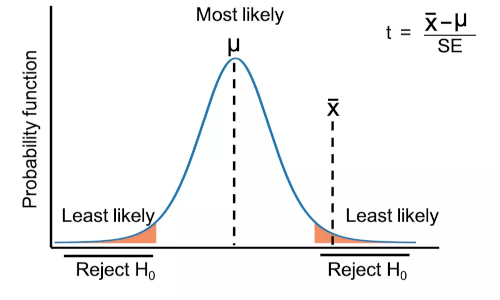## Perform three types of t-test in Python

Learn when to use t-test, types of t-test, assumptions, hypothesis, and formula for each type of test, and t-test calculation in Python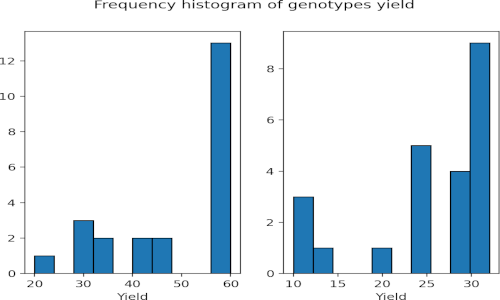## Mann-Whitney U test (Wilcoxon rank sum test) in Python

Learn when to use Mann-Whitney U test, assumptions, hypothesis, and formula, and test calculation in Python## Perform t-test from scratch in Python

Calculate three types of t-test from scratch## Reverse complementary

Reverse complementary of DNA sequences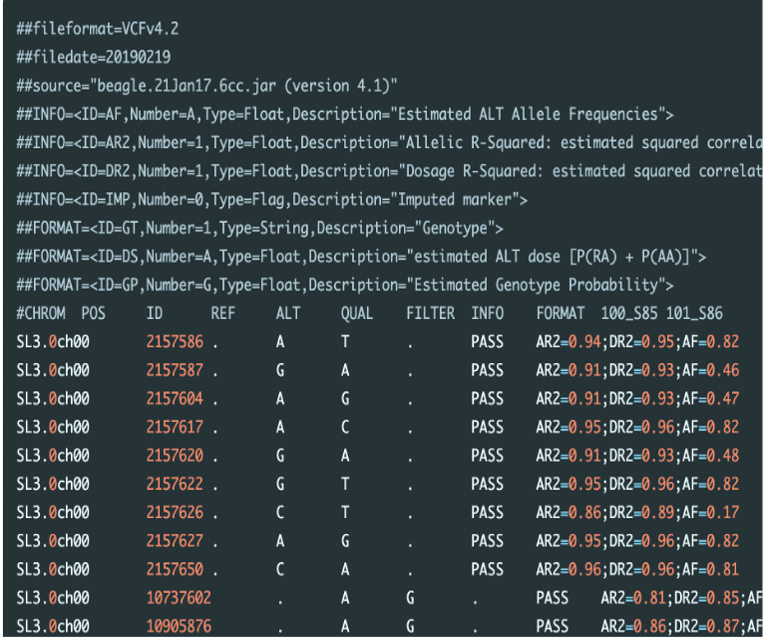## Concatenate and split VCF files

What is VCF file? VCF stands for variant call format It is a text file (file extension as .vcf) storing meta-information, marker and genotype data of ge...## Correlation analysis in Python

Correlation analysis using Python code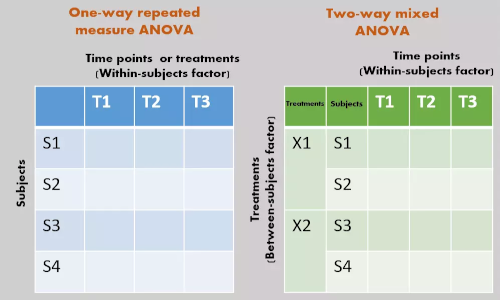## Repeated Measures ANOVA using Python and R (with examples)

Repeated Measure ANOVA in Python and R. This article explains repeated Measure ANOVA model, multiple pairwise comparisons, and results interpretation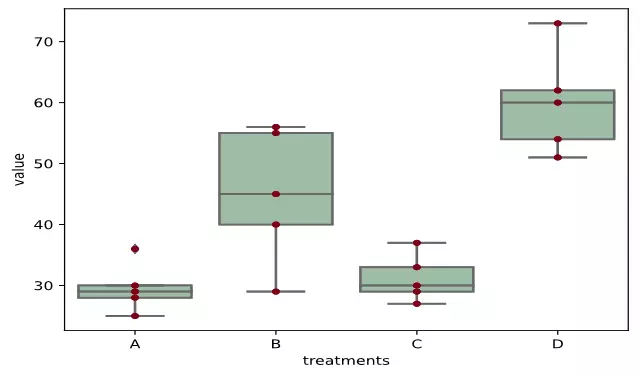## ANOVA using Python (with examples)

One and two-way ANOVA in Python. This article explains ANOVA model, formula, calculation, multiple pairwise comparisons, and results interpretation## Install and upgrade Python packages using pip on Windows, Linux, and Mac